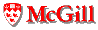# Memory Use Metrics

Last updated: December 4, 2002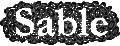For considering the memory use of programs, we concentrate on the amount and properties of dynamically-allocated memory (memory use for the stack is related to the call graph metrics, and memory for globals is not usually a dynamically varying value).

## Allocation Density Metrics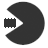The first metric required is just a simple value metric to measure how much dynamic memory is allocated by the program, per 1000 bytecode instructions (kbc) executed, and there are two variations.
• memory.byteAllocationDensity.value: Measures the number of bytes allocated per kbc executed. It is computed as the total number of bytes allocated by the program, divided by the (number of instructions executed / 1000).
• memory.objectAllocationDensity.value: Similar to the previous metric, but reports the number of objects allocated per kbc executed.
Although these metrics give a simple summary of how memory-hungry the program is overall, they do not distinguish between a program that allocates smoothly over its entire execution and a program that allocates only in some phases of the execution. To show this kind of behaviour, there are obvious continuous analogs, where the number of bytes / objects allocated per kbc is computed per execution time interval, and not just once for the entire execution (memory.byteAllocationDensity.continuous and memory.objectAllocationDensity.continuous).

## Object Size Distribution Metrics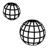• memory.averageObjectSize.value: The average size of objects allocated can be computed using the ratio of memory.byteAllocationDensity.value to memory.objectAllocationDensity.value. This metric is somewhat implementation dependent, as the size of the object header may be different in different JVM implementations.

Rather than just a simple average object size, one might be more interested in the distribution of the sizes the objects allocated. For example, programs that allocate many small objects may be more suitable for some optimizations such as object inlining, or special memory allocators which optimize for small objects.

• memory.objectSizeDistribution.bin: Object size distributions can be represented using this bin metric, where each bin contains the percentage of all objects allocated corresponding to the sizes associated with each bin. In order to factor out implementation-specific details of the object header size we use bin 0 to represent all objects which have no fields (i.e. all objects which are represented only by the header). In order to capture commonly allocated sizes in some detail, bins 1, 2, 3, and 4 correspond to objects using h+1 words, h+2 words, h+3 words and h+4 words respectively, where h represents the size of the object header. Then, increasingly coarser bins are used to capture all remaining sizes, where bin 4 corresponds to objects with size h+5...h+8, bin 5 corresponds to objects with size h+9...h+16, bin 6 corresponds to objects with size h+17...h+48 and bin 7 corresponds to all objects with size greater than h+48. Note that the sum of all bins should be 100%.

## Object Liveness Metrics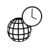Researchers interested in garbage collection are often interested in the liveness of dynamically-allocated objects. For example, the generational collection is potentially a good idea if a large proportion of objects have short lifetimes. For liveness metrics, time is often reported in terms of intervals of allocated bytes. For example, for an interval size of 10000 bytes, interval 1 ends after 10000 bytes have been allocated, interval 2 ends after 20000 bytes have been allocated and so on.

Object lifetimes can be estimated by forcing a garbage collection at the end of each interval, thus allowing one to find the amount of live memory after the collection, and to capture the death of objects that have become unreachable during that interval.

Based on the the amount of live memory at the end of each interval, we can compute the following two metrics.

• memory.highWaterHeapSize.value: This metric is computed as the maximum of all live memory amounts over all intervals.
• memory.averageHeapSize.value: This metric is computed as the average of the live memory over all intervals.

The highwater mark indicates how big the heap has to grow. The average tells us how big the heap is on average. If the average is much smaller than the highwater mark, then the program has some phase that is memory hungry, but other parts that are less memory hungry.

To compute interesting metrics about object lifetimes we define the birth_time of an object as the interval number in which it was allocated, the death_time as the interval number in which it was freed, and the last_used_time as the interval number in which the object was last touched. Each object then has a total_lifetime (death_time - birth_time), which is composed of two subintervals: active_lifetime (lastused_time - birth_time) and dragged_lifetime (death_time - lastused_time).

• memory.objectLifetime.bin: This metric reports the percentage of objects corresponding to each bin, where we have bins for lifetimes of 1, 2, 3, 4, 5...8, 9...16, 17...32 and greater than 32. If most objects have short lifetimes, then a generational collector may be useful. If many objects have very long lifetimes, then a collector that optimizes for long-lived objects may be preferred.

Another concept that is appearing in the garbage collection literature is the notion of the dragged objects. Dragged objects are those that are still reachable (live), but are never touched for the remainder of the execution. To define a metric that measures the amount of drag for a program we define the useful real estate for an object o as active_lifetime(o) x sizeof(o), the useless real estate for object o as dragged_lifetime(o) x sizeof(o), and the total real estate for o as total_lifetime(o) x sizeof(o). We can then look at total useless real estate as a fraction of total real estate. If this is a significant fraction, then dragged objects may be a problem in this benchmark.

• memory.uselessRealEstateFraction.value: This metric measures the overall useless real estate. It is computed as the sum of the useless real estate over all objects divided by the sum of the total real estate for all objects.
The previous metric summarizes the uselessRealEstateFraction for all objects. If this fraction is high, then there exists a drag problem in the benchmark. We can also categorize objects by their individual individual uselessRealEstateFraction values using bins.
• memory.uselessRealEstateDistribution.bin: For this metric we use 10 bins, one bin for each of the following intervals, 0:00...0:10, 0:11...0:20, ..., 0:91...1:00. Each bin counts the percentage of allocated objects with an individual uselessRealEstateFraction in that interval.